Next: Dyson Series Up: Time-Dependent Perturbation Theory Previous: Two-State System

# Nuclear Magnetic Resonance

Consider an atomic nucleus of spin one-half placed in a uniform-directed magnetic field, and then subjected to a small time-dependent magnetic field rotating in the-plane at the angular frequency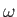. The net magnetic field is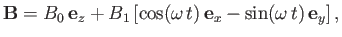(8.24)

where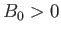and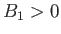are constants, with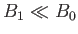. Assuming that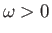, the rotating magnetic field represents the magnetic component of a left-hand circularly polarized electromagnetic wave propagating along the-axis . (Obviously, if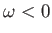then the wave becomes right-hand circularly polarized.) Now, we are only interested in the effect of the wave on the nuclear spin state, so we can neglect the wave's electric component. The magnetic moment of the nucleus can be written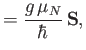(8.25)

where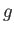is the (dimensionless) nuclear-factor,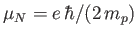the nuclear magnetron, and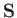the nuclear spin. (See Section 7.9.) Hence, the effective Hamiltonian of the system becomes(8.26)

where(8.27)

and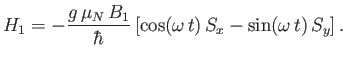(8.28)

The eigenstates of the unperturbed Hamiltonian are the spin up'' and spin down'' states, denoted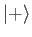and, respectively. (In other words, the eigenstates of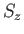corresponding to the eigenvalues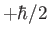and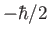, respectively.) Thus,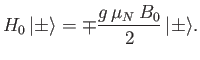(8.29)

The time-dependent perturbation to the Hamiltonian can be written(8.30)

where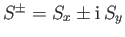are the conventional raising and lowering operators for spin angular momentum. (See Section 5.10.) It follows that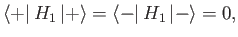(8.31)

and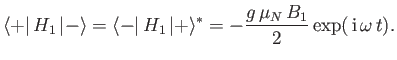(8.32)

Assuming that, it can be seen that this system is identical to the two-state system discussed in the previous section, provided that we make the identifications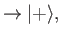(8.33)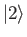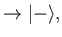(8.34)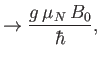(8.35)(8.36)

The resonant frequency,, is simply the precession frequency of the nuclear spin in a uniform magnetic field of strength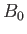. (See Section 5.6.) (Ifthen this precession is in the same sense as the direction of rotation of the magnetic component of a left-hand circularly polarized wave propagating along the magnetic field, and vice versa.) In the absence of the perturbation, the expectation values of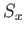and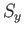oscillate because of the spin precession, but the expectation value ofremains invariant. However, if we apply a magnetic perturbation rotating at the resonant frequency (i.e.,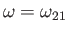) then, according to the analysis of the previous section, the system undergoes a succession of spin-flops,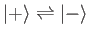, in addition to the spin precession. We also know that if the rotation frequency of the applied field is significantly different from the resonant frequency then there is virtually zero probability of the field triggering a spin-flop. The width of the resonance (in frequency) is determined by the strength of the rotating magnetic perturbation. In fact, the relative width of the resonance is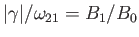. Experimentalist are able to measure the-factors of atomic nuclei to a high degree of accuracy by placing them in a strong magnetic field, and then subjecting them to a weak rotating magnetic field whose frequency is gradually scanned. By determining the resonant frequency (i.e., the frequency at which the nuclei absorb energy from the rotating field), it is possible to calculate the nuclear-factor, and, hence, the nuclear magnetic moment . In fact,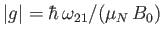. For a positive-factor, the resonance occurs when the weak magnetic field rotates in a left-handed sense (with respect to the direction of the strong magnetic field), and vice versa.Next: Dyson Series Up: Time-Dependent Perturbation Theory Previous: Two-State System
Richard Fitzpatrick 2016-01-22